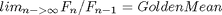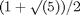## Loren on the Art of MATLABTurn ideas into MATLAB

Note

Loren on the Art of MATLAB has been archived and will not be updated.

# Programming for Multiple Datatypes

How do you write M-files in MATLAB that you would like to have work correctly whether the inputs are double precision or single? By correctly, I mean that the program gives an answer appropriate to the precision of the input.

### Special Constants and Functions in MATLAB to Help with Precision

There are some fairly new functions in MATLAB to help with precision, and some updates to existing functions. Let me first list some "constants" that might be interesting for working with different precision data:

• eps
• zeros, ones, Inf

There are several places in the documentation that are also particularly relevant:

And Cleve wrote this article for News and Notes in 1996 on floating point.

### Problem Description

Let's write a program that calculates a result either in single or double precision, depending on the input. Fibonacci numbers are a sequence of numbers with some interesting characteristics. As you calculate more numbers in the sequence, the ratio between successive Fibonacci numbers approaches the golden mean or golden ratio. In other words,My current web search found 42,000,000 pages for golden mean, 9,490,000 for golden ratio - wow!

### Golden Mean

The golden mean can expressed asWhat is its value, in double precision?

format long
GoldenMeanDouble = (1+sqrt(5))/2
GoldenMeanDouble =

1.61803398874989



GoldenMeanSingle = (1+sqrt(single(5)))/2
GoldenMeanSingle =

1.6180340



### How Many Fibonacci Terms Should We Compute?

How many terms in the Fibonacci sequence should we compute so that the ratio between successive terms doesn't change our estimate of the golden mean to within the appropriate precision? First, stop and take a guess for both double and single precision. Let's start with double, using the filter code from the previous blog to calculate the Fibonacci numbers.

format
nf = 100;
x = [1 zeros(1,nf-1)];
a = [1 -1 -1];
b = 1;
FibDouble = filter(b, a, x);

Calculate a few of the ratios and compare to the golden mean.

RatioDouble = FibDouble(2:11)./FibDouble(1:10)
ResidualDouble = FibDouble(2:11)./FibDouble(1:10) - GoldenMeanDouble
RatioDouble =

Columns 1 through 6

1.0000    2.0000    1.5000    1.6667    1.6000    1.6250

Columns 7 through 10

1.6154    1.6190    1.6176    1.6182

ResidualDouble =

Columns 1 through 6

-0.6180    0.3820   -0.1180    0.0486   -0.0180    0.0070

Columns 7 through 10

-0.0026    0.0010   -0.0004    0.0001



### Calculate Number of Terms

Here are the number of terms needed for double precision. Most people expect the number of required terms to be considerably higher.

numdouble = goldFibDouble
ResidDouble = FibDouble(numdouble)/FibDouble(numdouble-1) - GoldenMeanDouble
numdouble =

41

ResidDouble =

0



### Double Algorithm

Let's look at the algorithm. In this M-file, I do not worry about efficiency for calculating the Fibonacci sequence, but

type goldFibDouble
function nterms = goldFibDouble
% goldFib Number of Fibonacci terms to reach golden mean ratio.

fcurrent = 1;
fnext = 1;
goldenMean = (1+sqrt(5))/2;
tol = eps(goldenMean);  % Calculate increment for next closest number.
nterms = 2;
while abs(fnext/fcurrent - goldenMean) >= tol
nterms = nterms + 1;
temp  = fnext;
fnext = fnext + fcurrent;
fcurrent = temp;
end



### Floating Point Algorithm

What do I need to change in goldFibDouble to allow it to also calculate the number of terms for single precision? There are really only a few things I need to change. First, I probably want to calculate the Fibonacci numbers themselves in single, and the golden mean and tolerance. Finally, I need to change the function so I can specify what precision I want the output to be. Let's now look at goldFib where I made the necessary changes.

dbtype goldFib
1     function nterms = goldFib(dtype)
2     % goldFib Number of Fibonacci terms to reach golden mean ratio.
3
4     fcurrent = ones(dtype);
5     fnext = fcurrent;
6     goldenMean = (1+sqrt(cast(5,dtype)))/2;
7     tol = eps(goldenMean);
8     nterms = 2;
9     while abs(fnext/fcurrent - goldenMean) >= tol
10        nterms = nterms + 1;
11        temp  = fnext;
12        fnext = fnext + fcurrent;
13        fcurrent = temp;
14    end



### Number of Terms for Single and Double

Now let's see how many terms we need for both single and double.

numSingle = goldFib('single')
numDouble = goldFib('double')
numSingle =

19

numDouble =

41



You'll notice that on line 7 of goldFib, we calculate the relative accuracy for the golden mean in the correct precision. You can see the values here.

TolSingle = eps(GoldenMeanSingle)
TolDouble = eps(GoldenMeanDouble)
TolSingle =

1.1921e-007

TolDouble =

2.2204e-016



### Tools in MATLAB for Writing "Generic" Floating Point Programs

MATLAB has tools so you can create programs that work correctly on both single and double precision. You can see with this example that we only needed to modify a small number of lines and terms in the M-file in order to convert the file from handling double precision only to being able to handle both single and double precision calculations appropriately. Do you need to do something similar? Is it because you have large datasets and use single precision to help manage the memory? Let me know.

Published with MATLAB® 7.2